【GiantPandaCV导语】本文作为从零开始学深度学习编译器的番外篇，介绍了一下深度学习框架的Data Flow和Control Flow，并基于TensorFlow解释了TensorFlow是如何在静态图中实现Control Flow的。而对于动态图来说，是支持在Python层直接写Control Flow的，最后基于Pytorch介绍了如何将Python层的Control Flow导出到TorchScript模型以及ONNX模型。

# 0x1. DataFlow¶

#coding=utf-8

import os

def cal(a, b, c):
res = (a + b) * c
print(res)
return res

print(cal(1.0, 2.0, 3.0))


import tensorflow as tf

def cal(a, b, c):

init = tf.global_variables_initializer()
sess = tf.Session()

sess.run(init)
mul_op_res = sess.run([mul_op])

return mul_op_res

a = tf.constant(1.0)
b = tf.constant(2.0)
c = tf.constant(3.0)

print(cal(a, b, c))


Tensor("add:0", shape=(), dtype=float32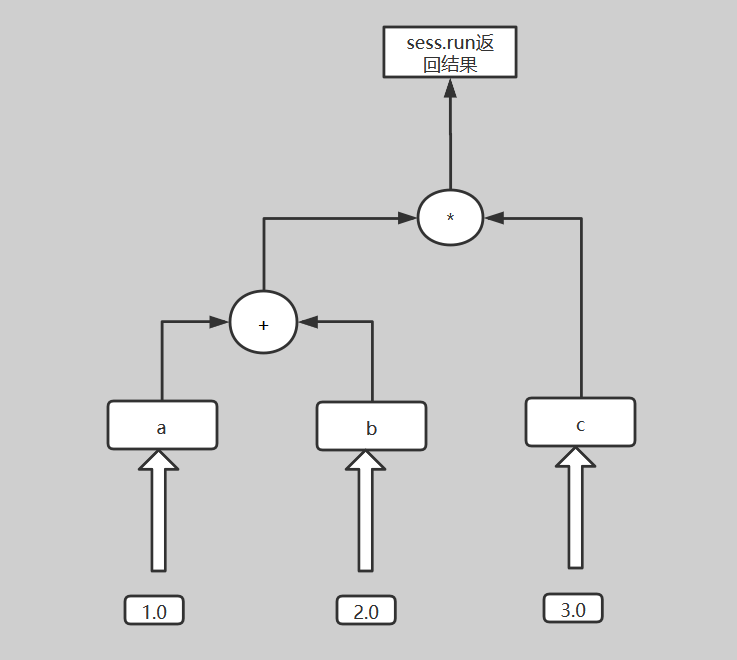# 0x2. Control Flow¶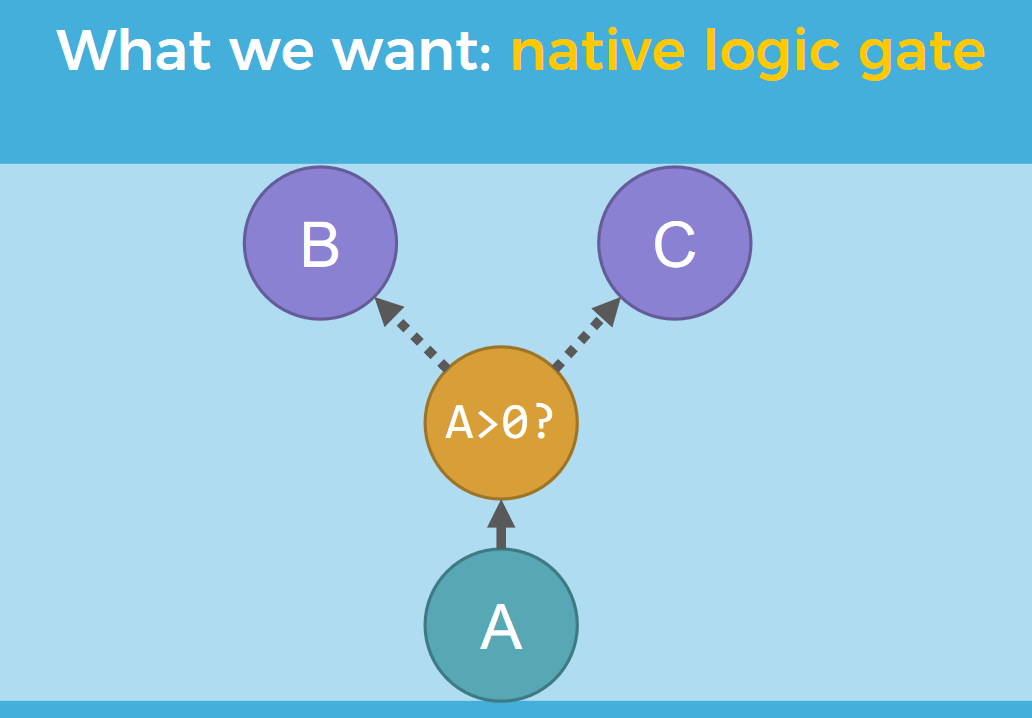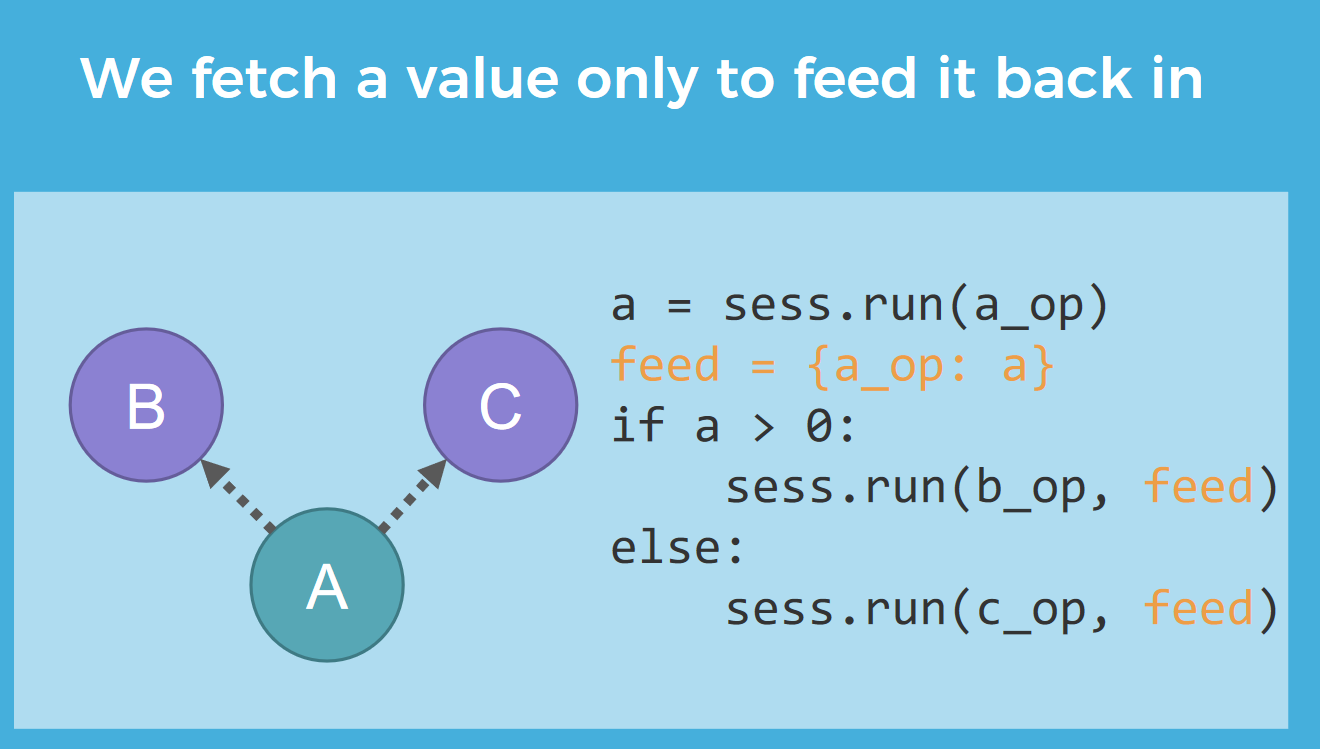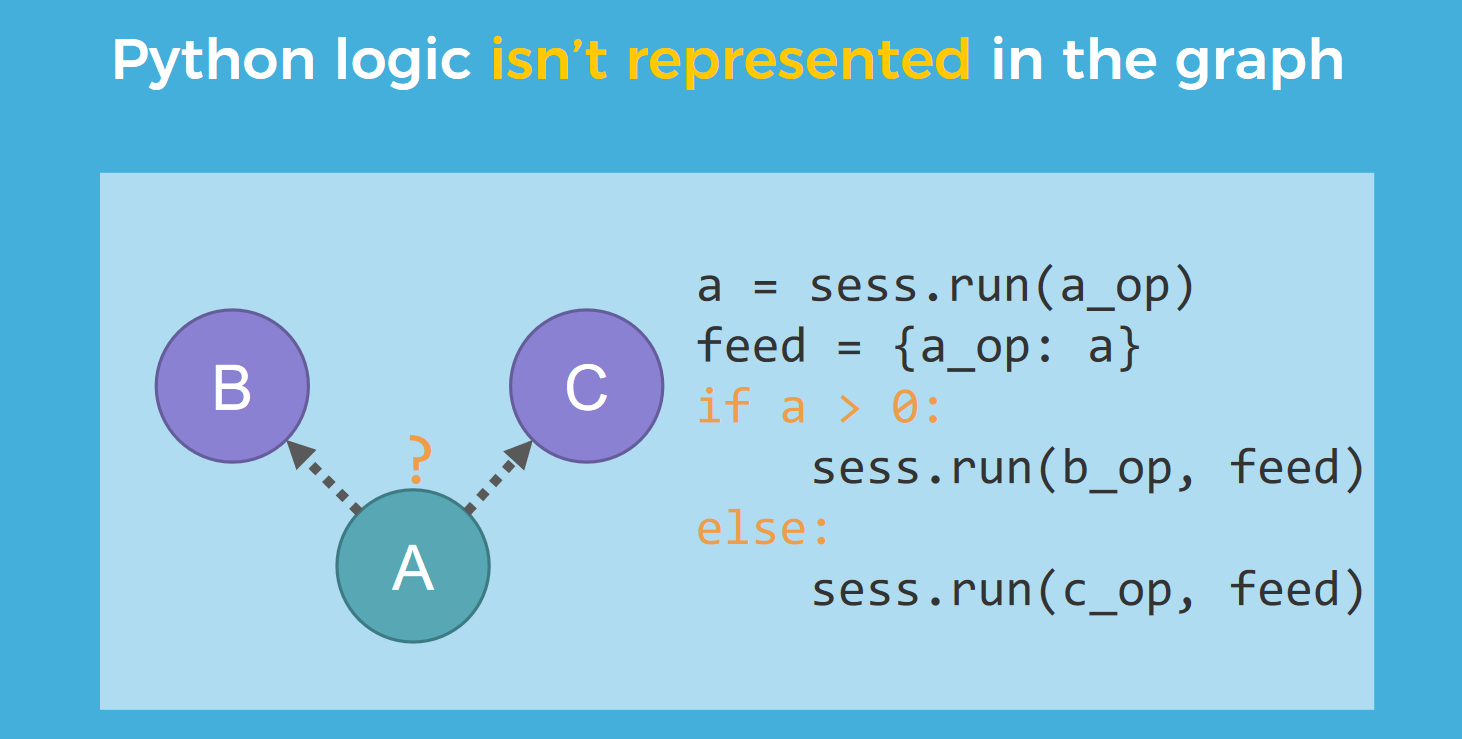Pytorch官方文档指出当我们导出ONNX的时候如果想导出Python层的控制流到计算图中就需要包一层@jit.script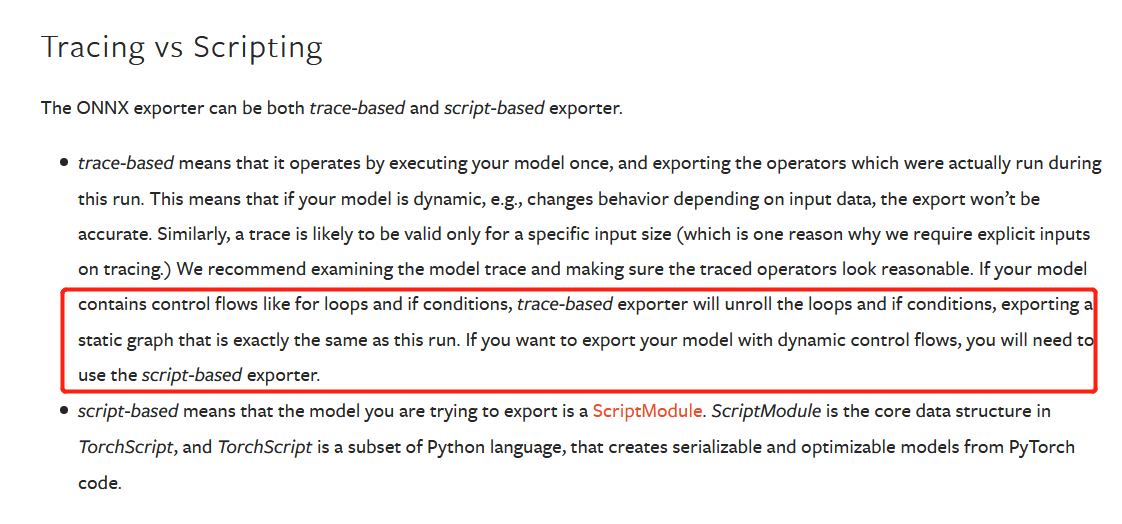## TensorFlow的原生控制流¶

TensorFlow提供了几个运算符用于原生控制流，如下：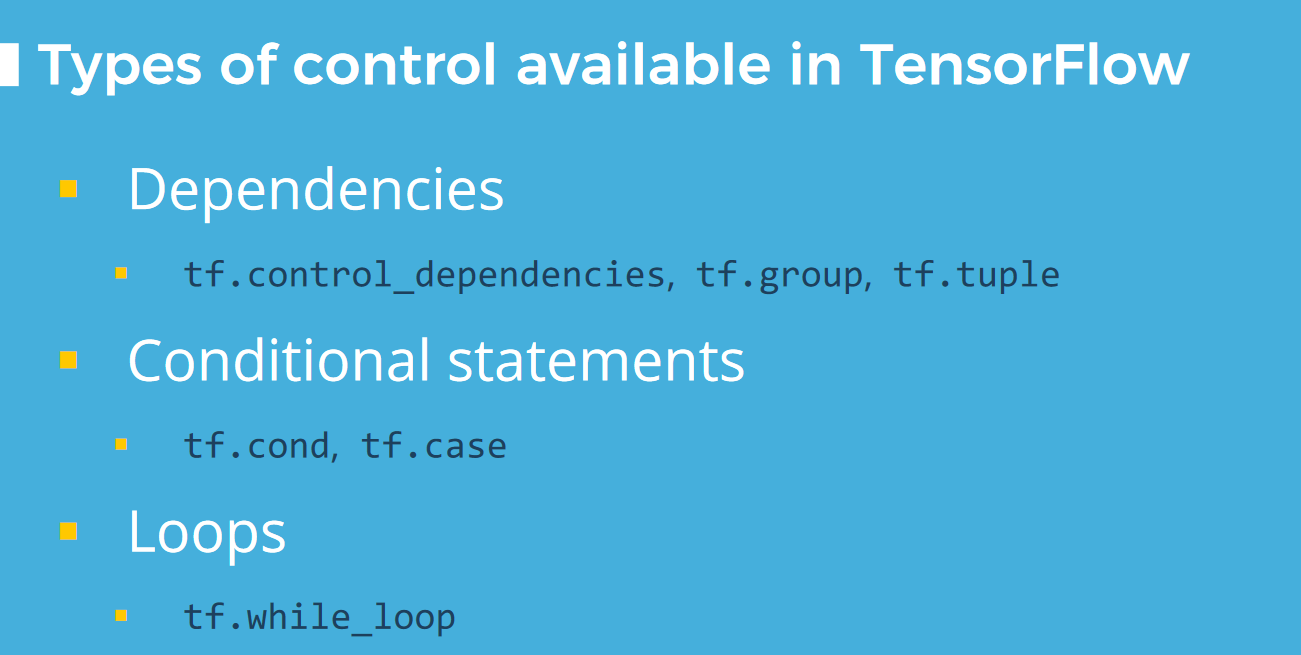• 高效。TensorFlow 计算图与 Python 交换数据比较慢，计算图如果是端到端的，才能将数据传输开销降到最低，运行速度更快。
• 灵活。静态计算图可以使用动态模块加强，计算图逻辑是自包含的。Pytorch目前比TensorFlow更受欢迎的主要原因就是前者为动态计算图，可以在运行时修改计算图。TensorFlow 利用控制流可以在一个静态定义的计算图中实现类似动态计算图的功能。
• 兼容。通过 TensorBoard 调试和检查计算图，无缝通过 TensorFlow Serving 部署，也可以利用自动微分，队列和流水线机制。

## 控制依赖¶

TensorFlow会记录每一个运算符的依赖，然后基于依赖进行调度计算。也就是说一个运算符当且仅当它的依赖都完成之后才会执行一次。任何两个完成依赖的运算符可以以任意顺序进行。但这种设定可能会引发竞争，比如：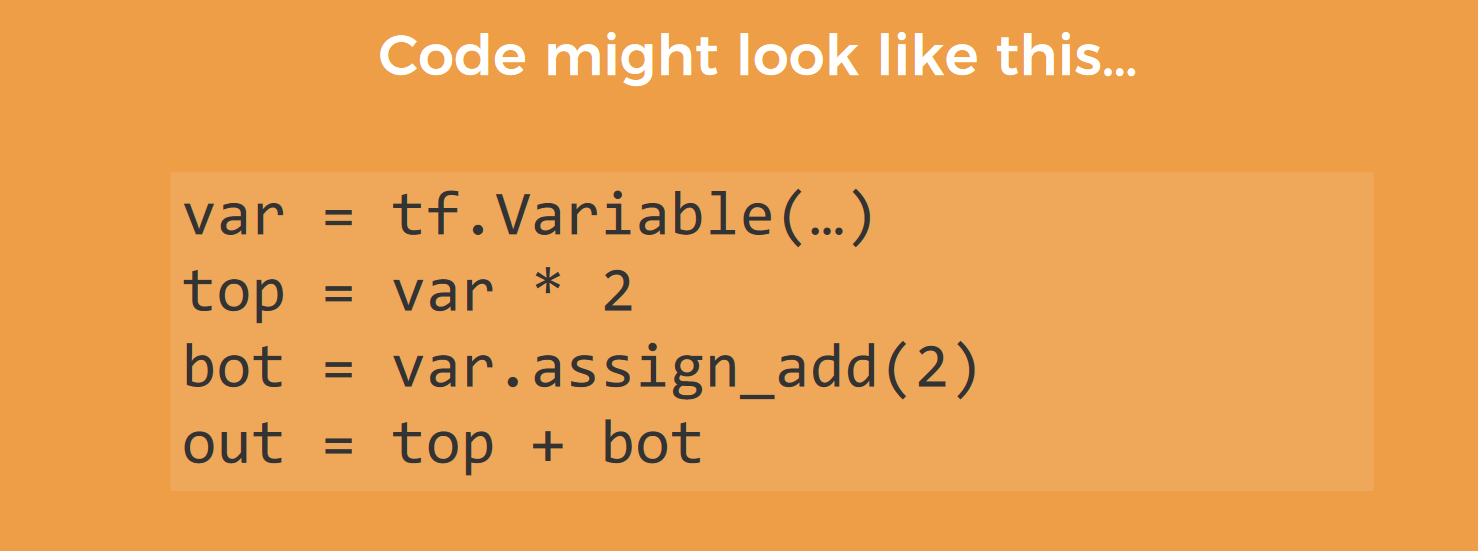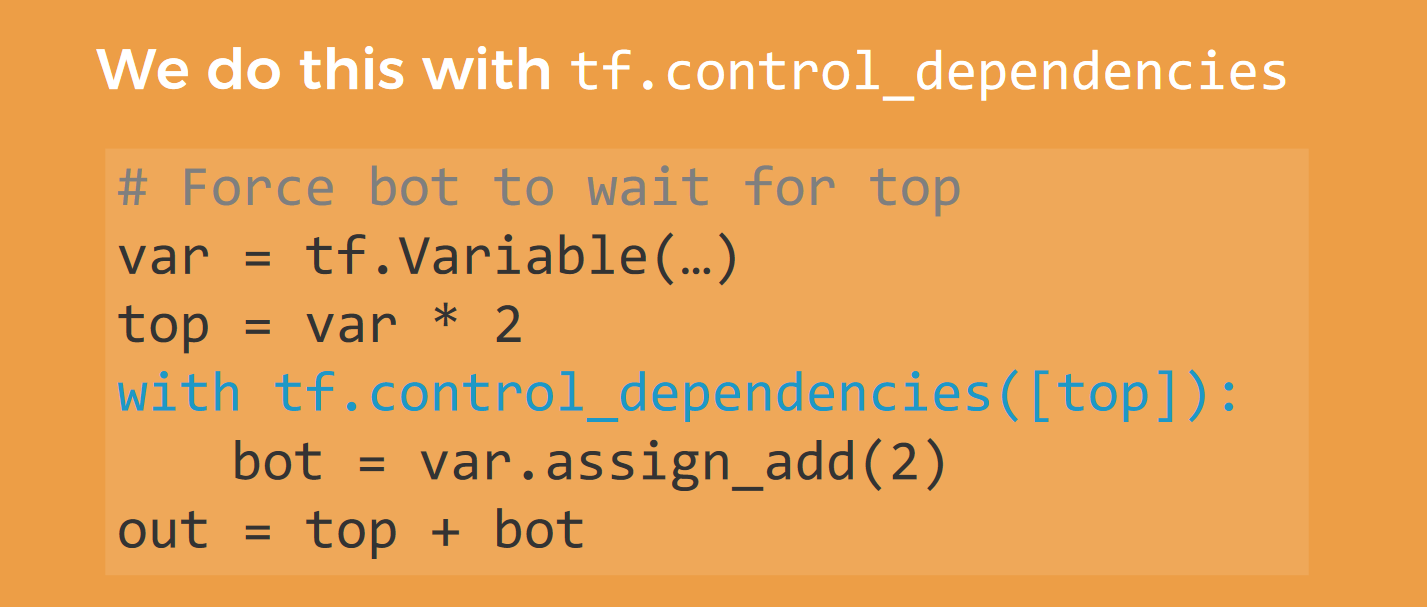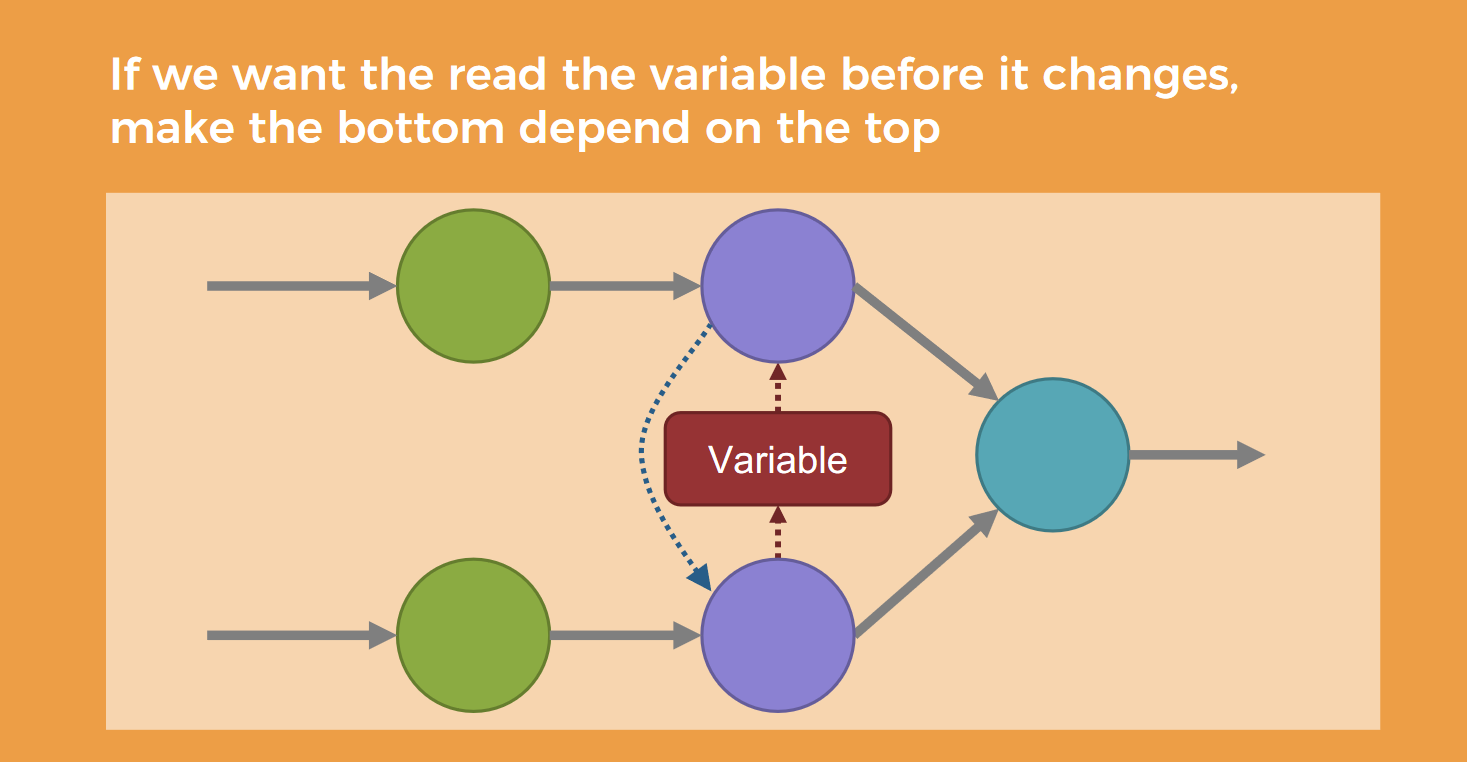## 条件分支¶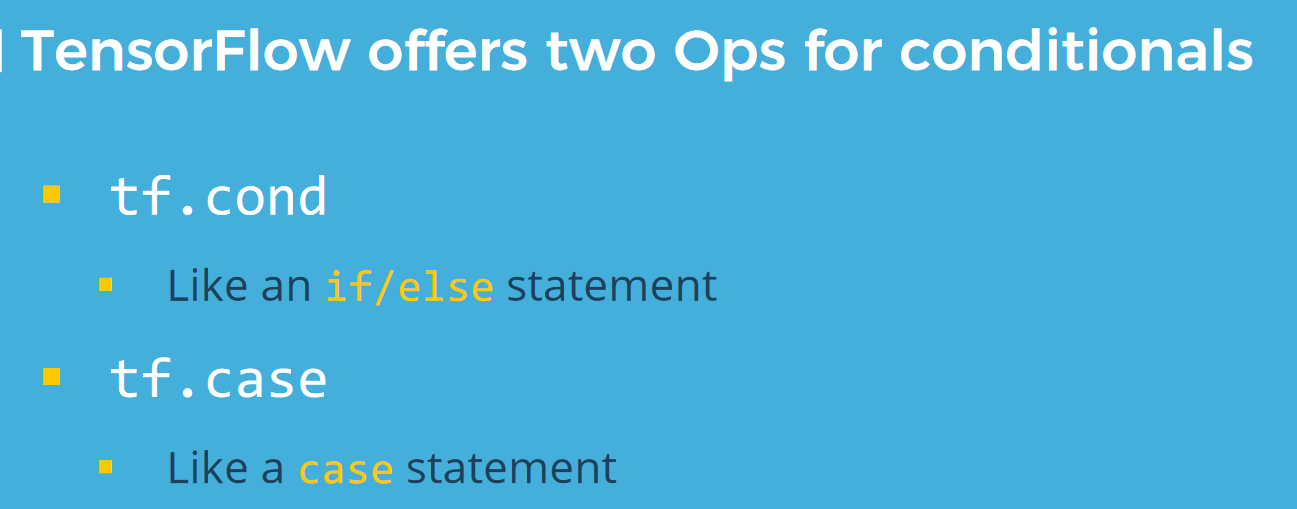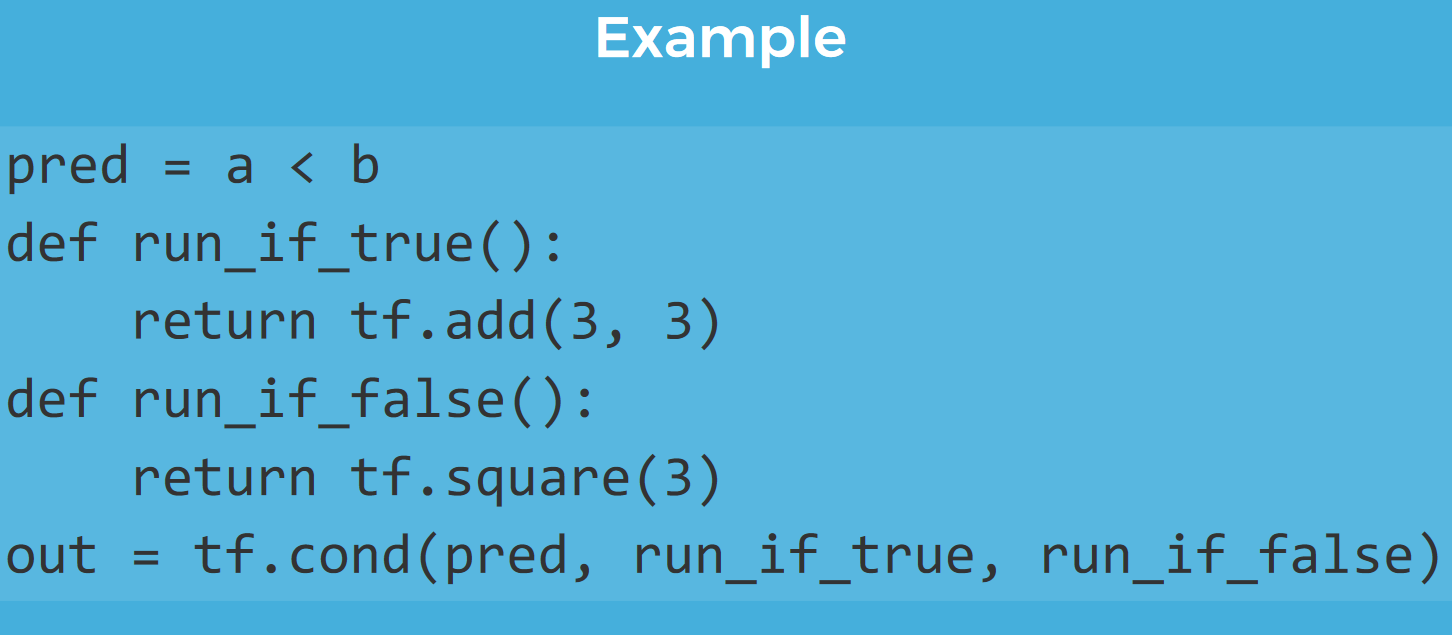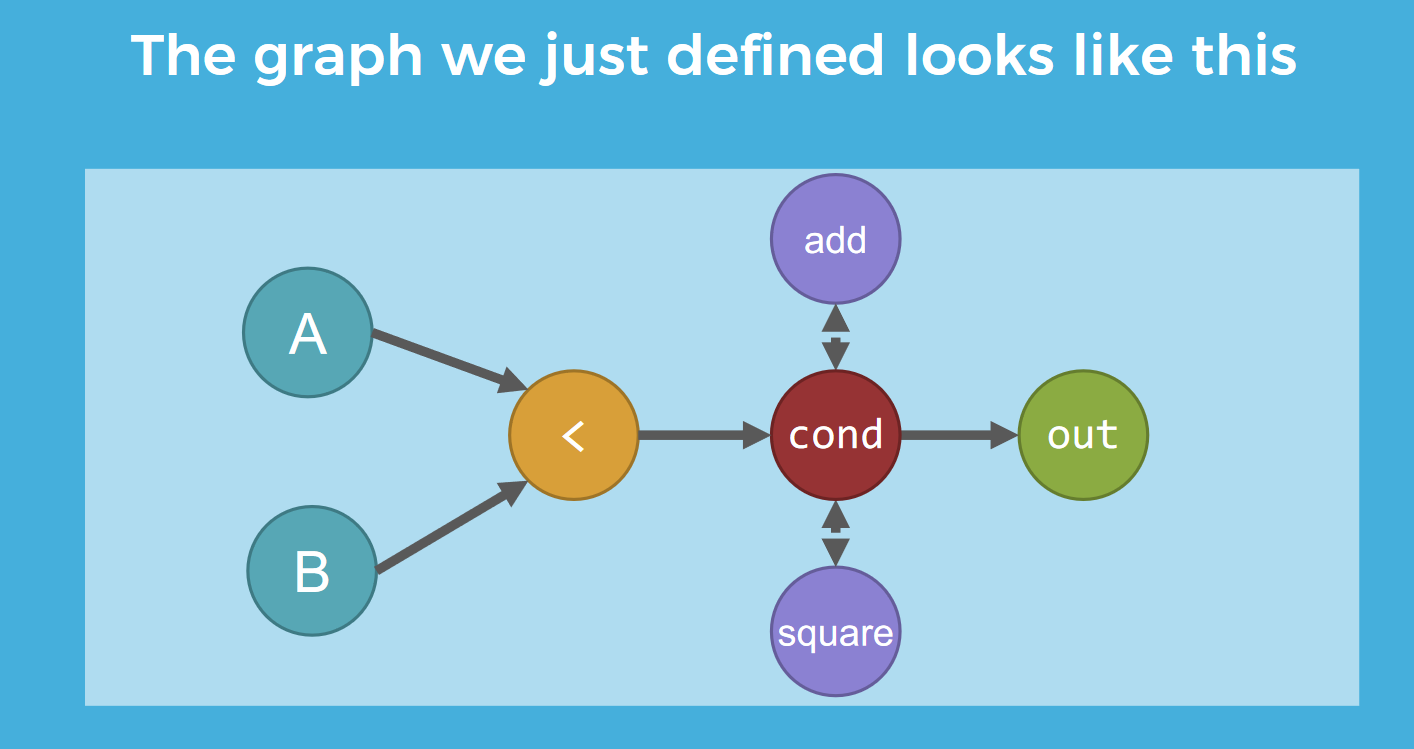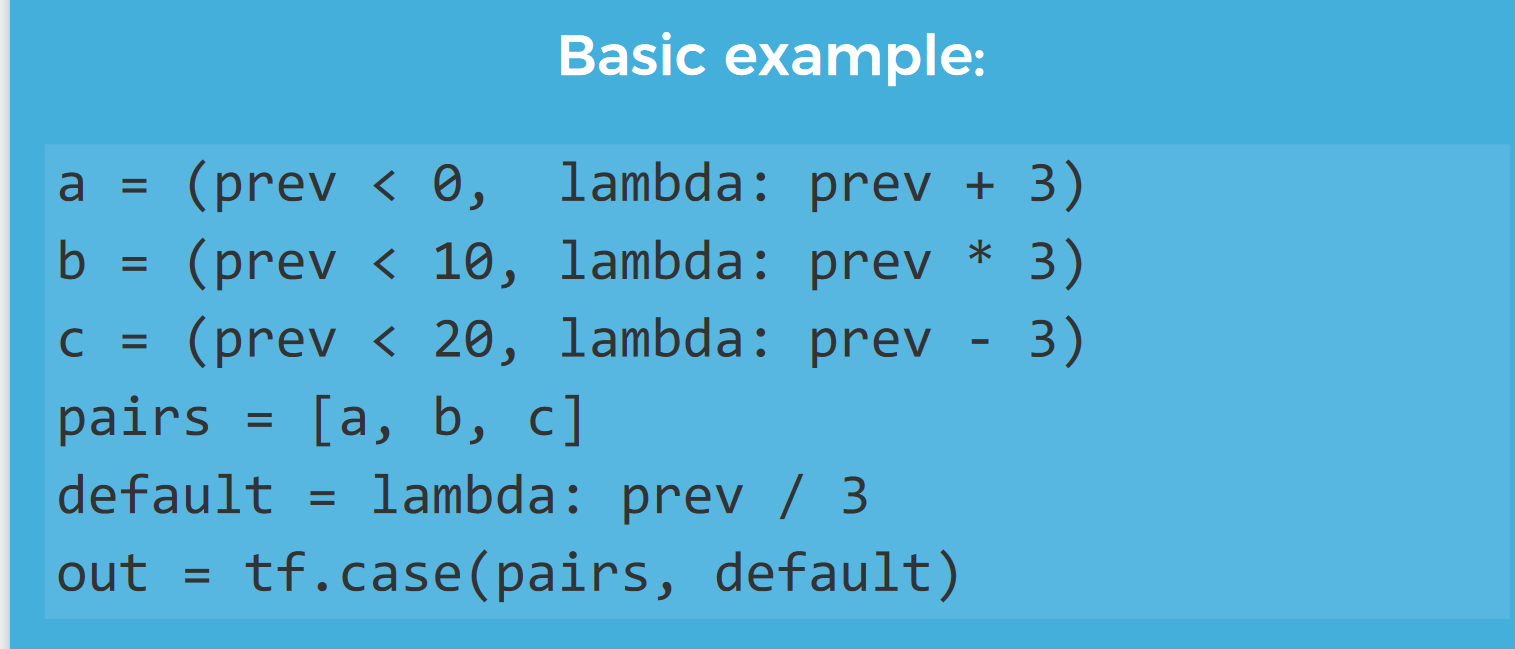## 循环¶

TensorFlow提供了tf.while_loop来构造循环块，感觉和RNN类似的结构有这个需求，例如：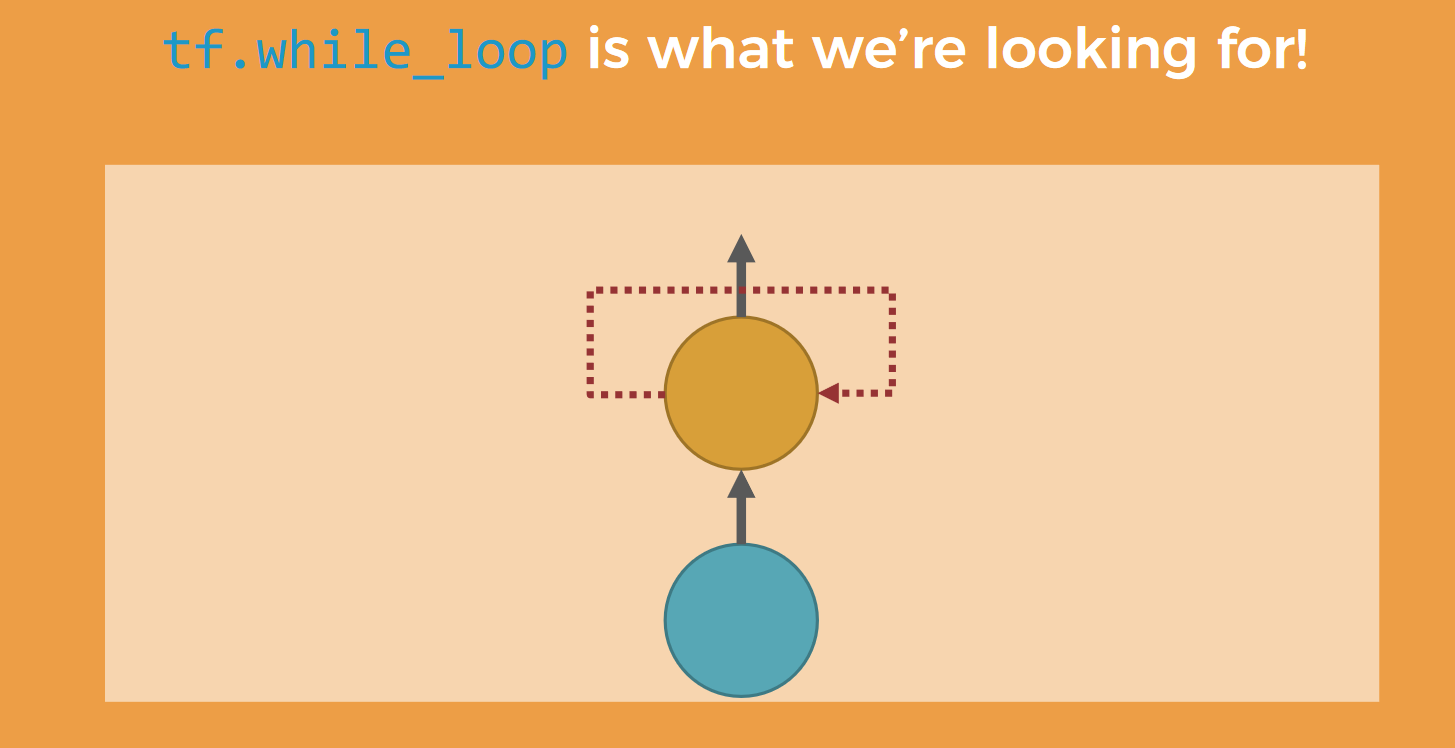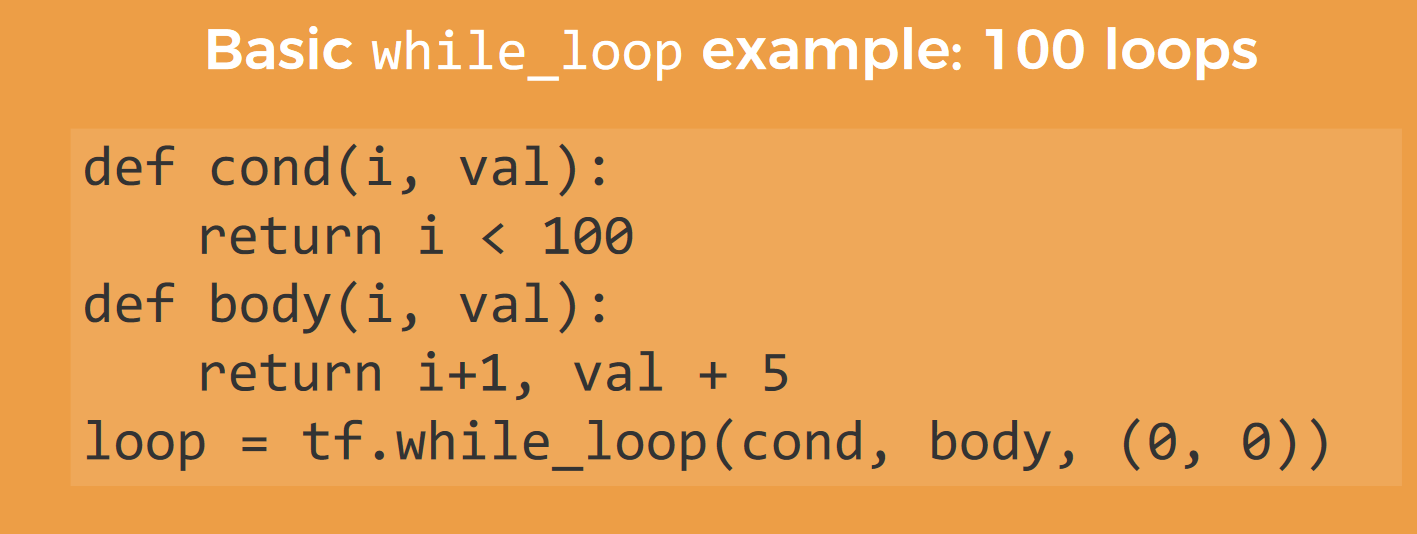# 0x3. Pytorch中的Control Flow¶

#coding=utf-8
import torch
import torch.nn as nn

class MyModule(nn.Module):
def __init__(self):
super(MyModule,self).__init__()
self.conv1 = nn.Conv2d(1,3,3)
def forward(self,x):
x = self.conv1(x)
return x

model = MyModule()  # 实例化模型
trace_module = torch.jit.trace(model,torch.rand(1,1,224,224))
print(trace_module.code)  # 查看模型结构
output = trace_module (torch.ones(1, 1, 224, 224)) # 测试
print(output)
# trace_modult('model.pt')


def forward(self,
input: Tensor) -> Tensor:
return (self.conv1).forward(input, )


#coding=utf-8
import torch
import torch.nn as nn

class MyModule(nn.Module):
def __init__(self):
super(MyModule,self).__init__()
self.conv1 = nn.Conv2d(1,3,3)
self.conv2 = nn.Conv2d(2,3,3)

def forward(self,x):
b,c,h,w = x.shape
if c ==1:
x = self.conv1(x)
else:
x = self.conv2(x)
return x

model = MyModule()

# 这样写会报错，因为有控制流
# trace_module = torch.jit.trace(model,torch.rand(1,1,224,224))

# 此时应该用script方法
script_module = torch.jit.script(model)
print(script_module.code)
output = script_module(torch.rand(1,1,224,224))


def forward(self,
x: Tensor) -> Tensor:
b, c, h, w, = torch.size(x)
if torch.eq(c, 1):
x0 = (self.conv1).forward(x, )
else:
x0 = (self.conv2).forward(x, )
return x0


import torch

# Trace-based only

class LoopModel(torch.nn.Module):
def forward(self, x, y):
for i in range(y):
x = x + i
return x

model = LoopModel()
dummy_input = torch.ones(2, 3, dtype=torch.long)
loop_count = torch.tensor(5, dtype=torch.long)

torch.onnx.export(model, (dummy_input, loop_count), 'loop.onnx', verbose=True)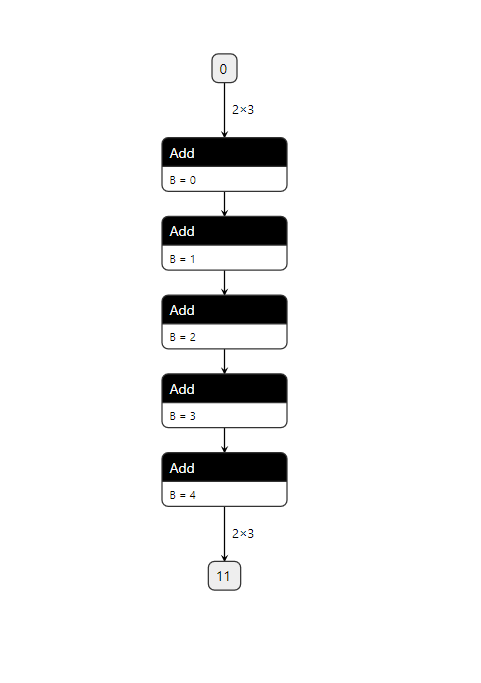import torch
# Mixing tracing and scripting

@torch.jit.script
def loop(x, y):
for i in range(int(y)):
x = x + i
return x

class LoopModel2(torch.nn.Module):
def forward(self, x, y):
return loop(x, y)

model = LoopModel2()
dummy_input = torch.ones(2, 3, dtype=torch.long)
loop_count = torch.tensor(5, dtype=torch.long)
torch.onnx.export(model, (dummy_input, loop_count), 'loop.onnx', verbose=True,
input_names=['input_data', 'loop_range'])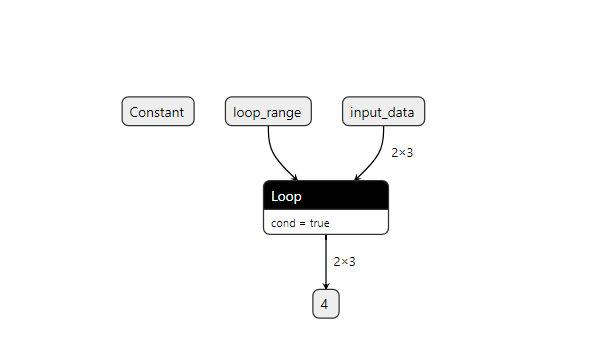# 0x5. 参考¶